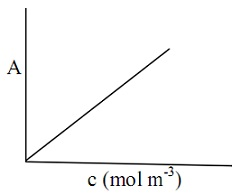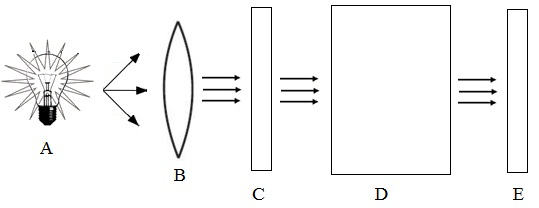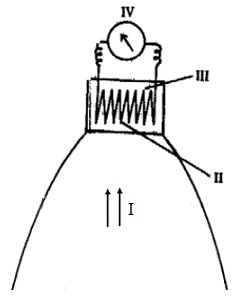#### Photochemistry, Chemistry tutorial

Introduction:

We are familiar that free energy should reduce for a physical or a chemical method to be feasible. This is applicable to thermal reactions (as well termed as dark reactions). The transformation of carbon-dioxide and water into starch is a reaction in which free energy rises. Under laboratory conditions, this reaction is not possible. However plants carry out starch synthesis by utilizing sunlight. Ozonization of oxygen and decomposition of ammonia are as well non-spontaneous reactions under the laboratory conditions. Though, these could occur by employing light of proper energy. These possibilities of latest reactions and new synthetic processes encouraged the scientists to probe to light-initiated reactions.

In photochemistry, we study the absorption and emission of light by matter. It comprises the study of different photophysical methods and photochemical reactions. Two significant photophysical methods are fluorescence and phosphorescence. Throughout fluorescence, light emission occurs in the presence of exciting radiation; however the emission stops once the exciting radiation is eliminated. In contrary to this, throughout phosphorescence, light emission occurs even after the elimination of the exciting radiation.

In photochemical reactions, the substances obtain the necessary activation energy during light absorption. Again, this is in contrary to the thermal reactions in which the reactants obtain their activation energy via collisions between the molecules.

Laws of Photochemistry:

Grotthuss-Drapper law and Stark-Einstein law are the two laws regarding the interaction between matter and light. Before illustrating these two laws, let us define the mathematical form of Beer-Lambert law.

Beer-Lambert law is helpful in computing the concentration of a solution on the base of its light absorption. This law associates the intensity of transmitted monochromatic light to the concentration of the solution and the thickness of cell in which the solution is kept.

The mathematical forms of Beer-Lambert law is represented below:

Log I0/I = A = ecl

Here, I0 = Intensity of the incident radiation

I = Intensity of the transmitted radiation

A = log I0/I = Absorbance or optical density of the solution

c = concentration of the solution deduced in mol m-3

I = thickness of the cell

€ = molar extinction coefficient; deduced in m2 mol-1

The molar extinction coefficient of a substance can be found out by employing a colorimeter or a spectrophotometer as follows. The various absorbance of a solution are measured at different known concentrations by using a cell of identified thickness (l). The plot of A against c provides a straight line (figure shown below) and its slope is equivalent to E1.Fig: The Graph of A vs C Plot

Therefore, e = slope/l

As l is known, e can be computed. By employing this e value, the concentration of a solution can be found out by measuring its absorbance.

Colorimeters and spectrophotometers are commercially available for the measurement of absorbance. In colorimeters, colour filters are employed to obtain the incident radiation in a specific wavelength range. Spectrophotometers encompass arrangements for acquiring almost monochromatic incident radiation. Both the colorimeters and spectrophotometers have devices for transforming the light transmitted into appropriate signal via detector devices. The signal produced from transmitted light is directly read as the absorbance values.

Grotthuss-Draper Law:

According to this law, only the light which is absorbed via a molecule can generate a photochemical change in it. This signifies that it is not adequate to pass via a substance to bring about a chemical reaction; however the light should be absorbed through it. Stark-Einstein law of photochemical equivalence gives a quantum mechanical form to Grotthuss-Draper law.

Stark-Einstein Law of Photochemical Equivalence:

Stark-Einstein law of the photochemical equivalence can be defined as follows:

Each and every molecule taking part in a photochemical reaction absorbs one quantum of radiation that causes the reaction.

Note: Stark-Einstein law is only applicable if the intensity of light is not extremely high.

This law is applicable to the main act of excitation of a molecule via light adsorption. This law assists in computing the quantum efficiency (F) that is a measure of the efficiency of the use of light in a photochemical reaction.

The quantum efficiencies for the formation of a product and for disappearance of the reactant are stated below:

The quantum efficiency for the formation of product (fR) = Number of molecules of product formed in 1 second/Number of quanta absorbed in 1 second

= (dNP/dt)/la

In the above equation, dNp/dt represents the rate of formation of the product. The units for dNp/dt are molecules per second. la signify to the number of photons absorbed per second. Likewise, we can state the quantum efficiency for the disappearance of a reactant.

The quantum efficiency for the disappearance of reactant (fR) = Number of molecules of reactant consumed in 1 second/Number of quanta absorbed in 1 second

= - (dNP/dt)/la

Where - dNR/dt is the rate of consumption of the reactant. The minus sign in - dNR/dt is because of the decrease in concentration of the reactant with time.

With respect to the Stark-Einstein law, each and every reacting molecule absorbs one quantum of light. Therefore,

The energy absorbed by one mole of substance undergoing Photochemical reaction = NAhv = NAhc/l

This unit of energy is as well termed as Einstein and as apparent from equation (NAhc/l) the value of Einstein mainly depends on v or l.

Here, NA = 6.022 x 1023 mol-1

h = 6.626 x 10-34 Js

c = 3 x 108 m s-1

l = Wavelength of the light in m unit

V = frequency in s-1 unit.

To compute the quantum efficiency, use the given steps:

i) Calculation of energy absorbed per Quantum:

Energy per quantum of radiation absorbed = hv = hc/l

ii) Calculation of la:

Generally intensity of light absorbed is represented in Js-1 units. From this, Ia could be computed by using the given equation:

Intensity in terms of number of photons absorbed in 1 second (l)

= Intensity in Js-1 units/Energy per quantum

Note:

Unit for energy per quantum = joule photon-1

Unit for l = joule second-1/joule photon-1

= photon second-1

iii) Calculation of -dNR/dt or dNP/dt:

At first, we have to get the number of moles of reactant consumed or of product made in 1 second by dividing the respective amount by the time in second unit.

In order to compute the rate of formation or rate of disappearance in molecule per second units, we have to make use of the given equations:

- dNR/dt = Number of moles of reactant Decomposed in 1 second x NA

As well, dNP/dt = Number of moles of product formed in 1 second x NA

iv) Calculation of Quantum Efficiency:

By using the equation (dNP/dt)/la) or equation - (dNP/dt)/la, fp or fR can be computed.

Experimental determination of Quantum Efficiency:

For the determination of quantum efficiency of a photochemical reaction, it is significant we assess the following:

• The rate of decomposition of the reactants or rate of formation of the products.
• The number of quanta absorbed in the specific time.

In concise, we require a process for measuring the amount of light absorbed, if we can work out a process for predicting the intensity of the incident light (lo) and the intensity of the light transmitted (l) via the reaction mixture, the intensity of the light absorbed (la) can be computed by employing the relationship;

Ia = lo - l

For measuring lo and l, similar cell is employed. Io corresponds to the measurement of intensity with empty cell (termed as blank) and l, to the measurement by using the cell having the reaction mixture.

The experimental set-up employed for measuring the intensity of light is illustrated in the figure shown below.

As a source (A) of visible light, a high intensity tungsten lamp or quartz halogen lamp or xenon arc lamp can be employed. For getting ultraviolet light, hydrogen or deuterium lamps are excellent sources. The light is first passed via a lens, B (that is, to obtain a parallel beam of light) and then via a Monochromator (C). The Monochromator results a narrow band of light in the desired wavelength. The monochromatic light then passes via the reaction cell (D). The light transmitted through the reaction cell reaches the detector (E).Fig: Intensity of Light during a Photochemical Reaction

Note:

• While employing ultraviolet light as a source of radiation, quartz cuvettes (that is, cells) and lenses should be employed.
• As Pyrex glass absorbs light beneath 300 nm, it can be employed for radiation above 300 nm only.
• The visible light consists of the wavelength region, 800 to 380 nm. The ultraviolet light consists of wavelength beneath 380 nm and above 199 nm.

The detector is employed for measuring the intensity of light. The quantitative measurement of light intensity mainly based on the energy conversion or on chemical reaction is known as actinometry. Detectors like thermopiles and the photoelectric cells function on energy conversion principle.

Thermopile:

Thermopile (figure shown below) is a device helpful for transforming light into heat, and then, heat to electricity. It consists of a set of junctions of various metals having a blackened surface. This black coating is to make sure absorption of all radiation falling on it. The energy so absorbed rises the temperature of the metals. Thermopile transforms this temperature increase to a potential difference. Light intensity is associated to electricity produced by it. The detector device should be calibrated against a standard light source. Thermopile could be employed as a detector for light of any wavelength.

Note:

Thermopile comprises of thermocouples joined in series and it produces electricity on heating.Fig: Thermopile

Thermopile: (I) Light radiation (II) Blackened surface exposed to radiation (III) Unexposed surface at the back (IV) Galvanometer

Photoelectric Cells:

Photoelectric cell transforms light directly to electricity. The current produced is directly proportional to the light intensity. Photoelectric cell is the evacuated bulb by a photosensitive cathode. Light radiation yields in the emission of electrons from the cathode and then collected at the anode. Therefore, the flow of current is caused. The photoelectric cell is susceptible to the wavelength of the light employed and should be calibrated by employing a thermopile.

Chemical Actinometers:

Light intensity could be predicted through a quantitative estimation of the substance made or decomposed by light. The photochemical decomposition of K3Fe (C2O4)3 is a broadly employed chemical process for estimating the intensity of light between the wavelength range 250 nm and 577 nm. Light in this wavelength range causes K3Fe(C2O4)3 dissolved in the sulphuric acid to react as follows:

2Fe3+ + 2e- → 2Fe2+

C2O42- → 2CO2 + 2e-

The concentration of Fe2+ is expected calorimetrically via complex formation by 1,10-phenanthroline. This complex consists of a deep red colour. The concentration of the complex is equivalent to the concentration of Fe2+ present before Complexation takes place.

The Quantum Efficiencies of some reactions:

Whenever a photochemical reaction obeys Stark-Einstein law, then 'f' should be unity. The studies on a large number of photochemical reactions point out that as some of them follow the Stark-Einstein law, most of the others don't. The quantum efficiencies of few photochemical reactions all along with their efficient wavelengths are illustrated in the table shown below.

Reaction                     Effective wavelength/nm     f

2NH3 → N2 + 3H2               Ð 210                      0.2

2NO2 → 2NO + O2              Ð 366                      0.7

H2 + Br2 → 2HBr                Ð 511                      0.01

H2S → H2 + S                     Ð 208                      1.0

2HI → H2 + I2                     Ð 327                      2.0

3O2 → 2O3                          Ð 175                      3.0

H2 + Cl2 → 2HCl                 Ð 478                      106

The photochemical reactions for which the quantum efficiency is a small integer are stated to obey Stark-Einstein law. Illustrations are the dissociation of H2S or Ozonization of oxygen and so on. Reactions similar to the formation of hydrogen chloride or hydrogen bromide don't obey Stark-Einstein law. The quantum efficiency for the former reaction (H2 + Cl2 → 2HCl) is extremely high whereas it is much low for the latter (H2 + Br2 → 2HBr). In order to describe such discrepancies, Bodenstein indicated that photochemical reaction comprised two different methods: the primary and the secondary methods.

a) In the primary method, absorption of one quantum of light yields in the excitation of one molecule or an atom (A) to manufacture the excited species, AD

Atom or molecule    Excited species

b) In the secondary method, the excited species experiences a chemical change

A → Products

According to the Bodenstein, the deviations from Stark-Einstein law are because of the secondary methods.

In case of reactions including low quantum yields, the number of molecules decomposed via the absorption of one quantum of radiation is less than one. The possible cause for the low quantum yields are described below:

• The excited species made at the first step might be deactivated via collisions before the product could be made. This method is known as quenching. At times, the presence of paramagnetic substances such as oxygen or nitric oxide could yield in quenching leading to the low quantum result for a chemical reaction.

• One or more of the reactions in the secondary or primary methods might be endothermic. High energy need could reduce the reaction rate. The quantum result for the formation of hydrogen bromide is low because of the endothermic nature of the reaction between Br and H2.
• The excited species might re-join to provide the reactant molecule. In the hydrogen bromide formation, the other reason for low quantum result is the recombination of bromine atoms that are manufactured in the primary step.

In case of reactions having high quantum results, the excited species made in the first step could initiate a sequence of chain reactions. This causes a huge number of molecules to react via absorption of one quantum of light, as in the case of manufacture of hydrogen chloride.

Tutorsglobe: A way to secure high grade in your curriculum (Online Tutoring)